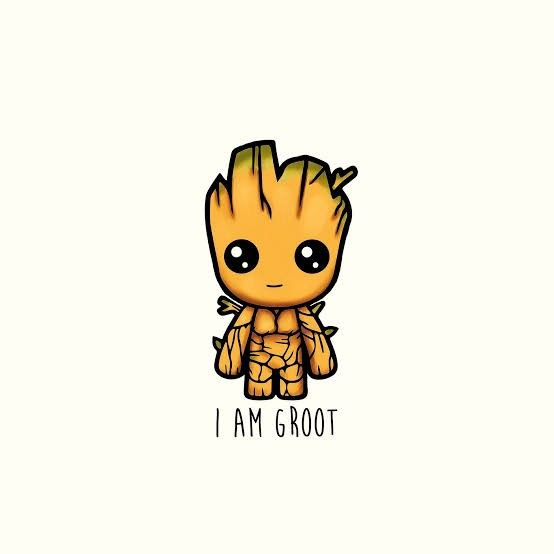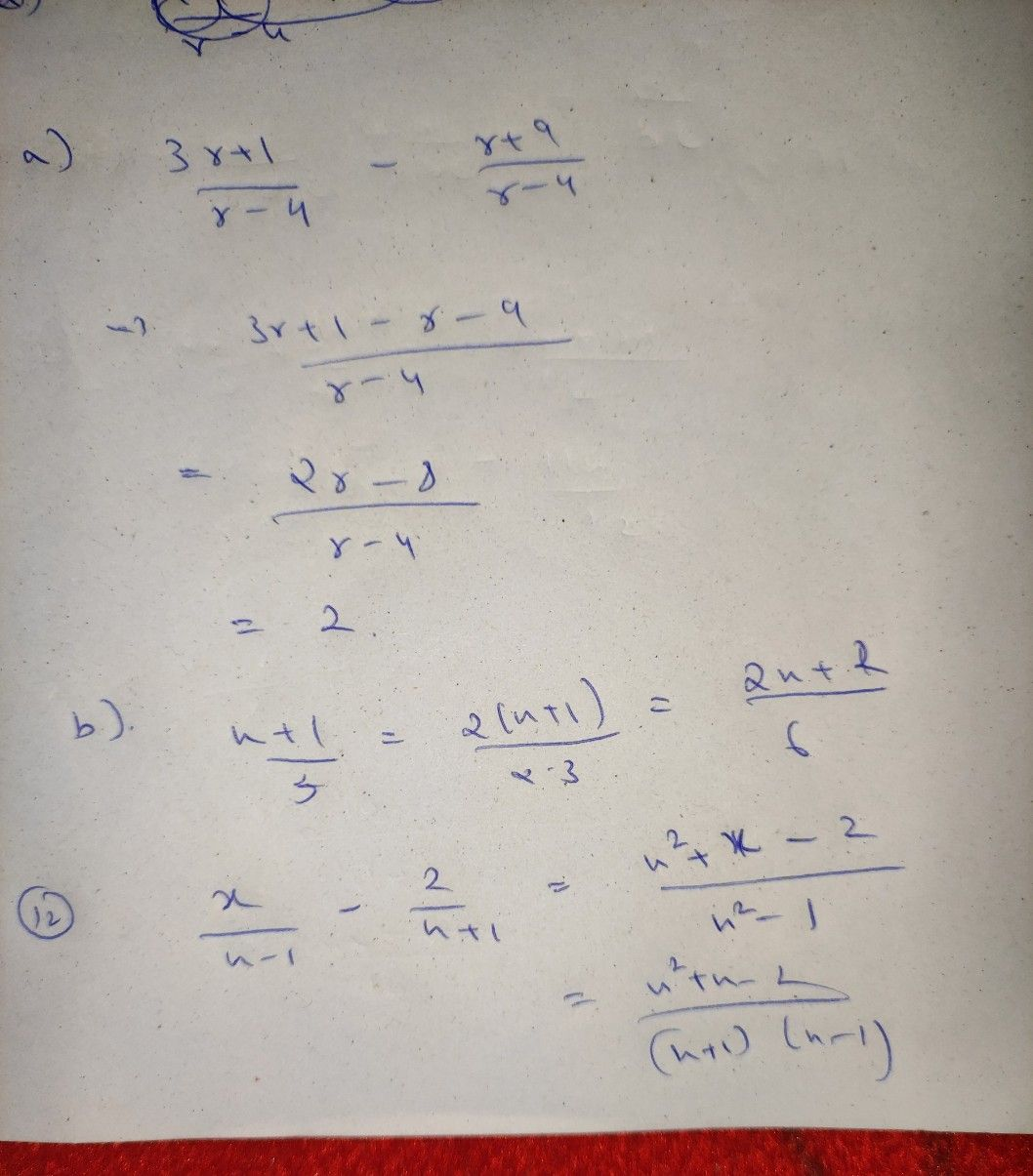Symbol
Problemr+9 $3r+1$ 9. Subtract r-4 from r-4. A. 2 C. 6 B. 4 D. 8 $10.4$ Using the $LcD6$ look for the equivalent rational algebraic expression of $\dfrac {x+1} {3}$ A. 2x+1 6. 2x+2 C. 6x+1 D. 6x3. +6 11. $Look$ for the equivalent rational algebraic expression of each of a+a 1 and b+1 b if the $LCD$ is ab. A. $\dfrac {ab+1} {ab},\dfrac {ab+b} {ab}$ C. $\dfrac {ab+b} {ab}$ $\dfrac {ab+a} {ab}B$ $\dfrac {ab-a} {ab},\dfrac {ab-1} {ab}$ D. $\dfrac {ab-b} {ab},\dfrac {ab-a} {ab}$ $pliy^{\dfrac {x} {x-1}-\dfrac {2} {x+1}}$ $12.y$ Write as one fraction and simplifyx-1 $A.$ $\dfrac {x^{2}+x+2} {\left(x-1\right)\left(x+1\right)}$ C. $\dfrac {x^{2}-x-2} {\left(x-1\right)\left(x+1\right)}$ B. $\dfrac {x^{2}-x+2} {\left(x-1\right)\left(x+1\right)}$ D. $\dfrac {x^{2}+x-2} {\left(x-1\right)\left(x+1\right)}$
7th-9th grade
Algebra
Search count: 108
SolutionQanda teacher - Anwesha